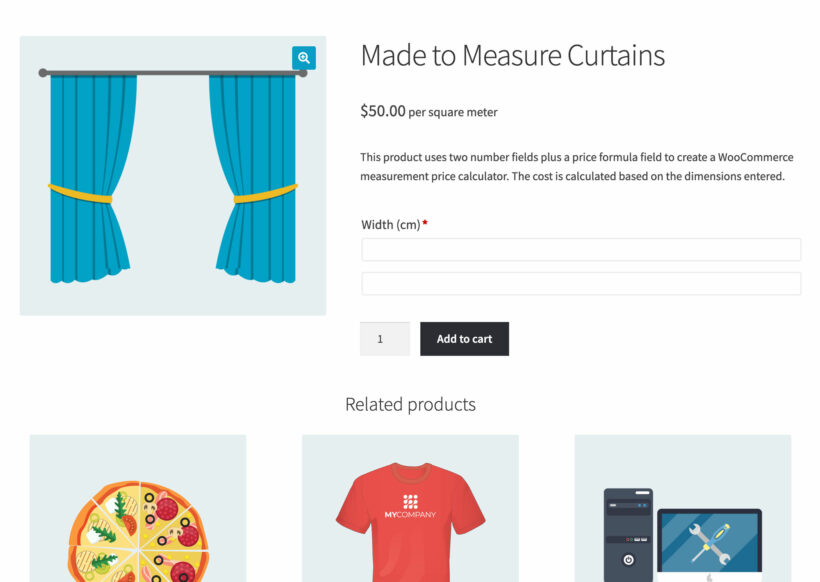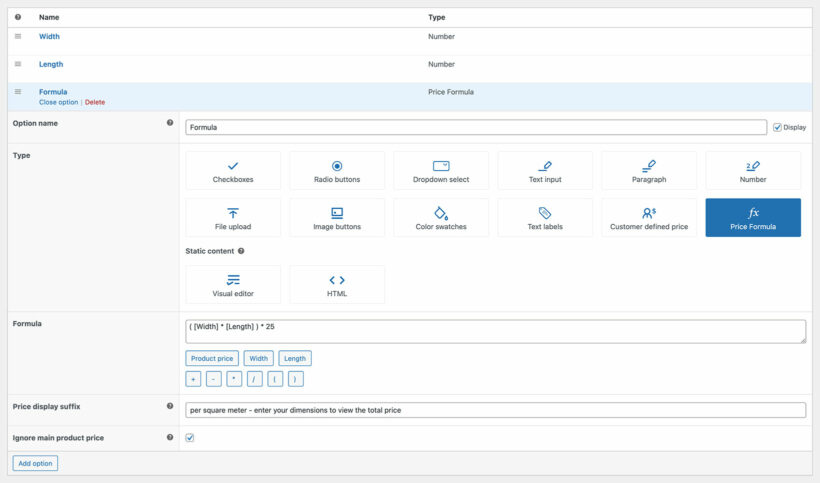# How to create a price formula for your WooCommerce products

The WooCommerce Product Options plugin comes with a Price Formula field type which you can use to create a product price calculator. This calculates a custom price for the product based on the data which the customer enters in your other product options.

## How it works (an example)Imagine that you're selling made-to-measure curtains which are charged based on the area of fabric ordered. To achieve this, you can create a product with the following options:

• Width (number field)
• Length (number field)
• Price Formula

The price formula field will perform a calculation which multiplies the width, length, and the cost per meter. This will then update the total price of the product.

You can create very simple or very complex price formulas, depending on your requirements. For example, if you're selling curtains then you might want to:

• Add a fixed base cost which will be added to the calculated price regardless of the curtain dimensions.
• Double the calculated price because you're selling the curtains as a pair.

You can also choose whether or not to include the main product price in the calculated price. For example, you might have entered the main price for the curtains as \$200 so that you can display it as "From \$200" on the product page, but you may not want to add this to the calculated price.

## Instructions

To create a price formula, go to Products → Product Options, edit the option group that you want to add the calculation to, and create the following options:

• One or more Number fields. Do not add pricing to these because that will be handled by the Price Formula field.
• A Price Formula field.

Enter the formula which will be used to calculate the price. You can use the following variables and operators. These are displayed underneath the Formula field so that you can click to add them as required:

• Product price - use this to optionally add the main price of the product to the formula, regardless of whether 'Ignore main product price' is enabled below. This is the price for the product or variation which you entered on the 'Add/Edit Product' page.
• Each of the Number fields for the product (these need to be added in square brackets, e.g. [Width] or [Length]).
• + - * and / - use these to add mathematical calculations to the formula, for example to multiple two number fields or add the main product price.
• ( ) - use brackets to tell the formula which part of the calculation to make first.

## How should I use brackets in a formula?

Brackets are designed for multi-step calculations and tell the price formula which part of the calculation to perform first. Let's illustrate this with an example of a product where the customer has entered the width as 5 and the length as 10:

• The formula `[Number 1] * [Number 2] + 50` is unclear and could lead to errors because there's nothing to tell it whether to multiply Number 1 by Number 2 and then add 50 to the result; or to add 25 to Number 2 and then multiply the result by Number 1. This gives you several possible answers.
• The formula `([Number 1] * [Number 2]) + 50` is better because the brackets tell it to multiply Number 1 by Number 2 first, and then add 50 to the result. This means that there is only one possible answer and avoids any errors.

## A simple exampleThe following price formula is quite simple: `([Width] * [Length]) * 25`

First, it multiples two number fields - width and length. It then multiplies the total by 25 to get the final price.

For example, you might use this for products which you are selling by the square meter.

This price formula is more complex: `((( [Width] * [Length] ) /10000) * 25) * 2`

It might be used to sell a pair of by the meter, where the customer enters the dimensions in centimetres but the fabric is priced at \$25 per square meter - so the formula needs to convert the centimetres into metres!

It uses three sets of brackets to perform each part of the calculation in the correct order. Let's break it down:

1. The innermost set of brackets (blue) contains [Width] * [Length]. This means that the formula needs to multiply the width by the length first in order to calculate the total area.
2. The next set of brackets (red) tells the formula to divide the total area (as calculated in step 1) by 1,000. This converts the area from centimetres into metres.
3. Next, the green brackets multiply the number by 25 - i.e. the price per meter.
4. Finally, everything is multiplied by two because the curtains are sold in pairs, but the customer has only entered the dimensions per curtain.

As you can see, price formulas can be complex and it's important to break them into stages to avoid mistakes.

## My formula is calculating the wrong price!

These are the most common reasons for a formula not working correctly:

• Have you made a mistake in the formula? Price formulas can be complex, especially if you are adding lots of parts to the equation. If you're not confident with the math then we recommend asking an expert to check it for you.
• Is the main product price being added to the total? WooCommerce requires you to set a price for the product or variation, otherwise it will not be purchasable. You do this on the 'Add/Edit Product' page. Normally, WooCommerce will add this to the total cost of the product, even if you are using a price formula field. You can stop this from happening by ticking the 'Ignore main product price' box in the Price Formula settings.
• Are you using brackets correctly? Brackets can make or break a formula, so check these carefully. Remember - the parts of the formula in brackets will be calculated first. Learn more about how to use brackets.